Next: Theory Up: Isospin mixing in nuclei Previous: Isospin mixing in nuclei

# Introduction

The atomic nucleus is a quantum system composed of the two types of strongly interacting fermions, the nucleons. The charge independence of the nuclear interaction is at the roots of the isospin symmetry [1,2]. This concept remains valid even in the presence of Coulomb interaction, which is the major source of the isospin breaking. This is so because of the smallness of the isospin-breaking isovector and isotensor components of the Coulomb field as compared to the isospin-conserving components of the nuclear and Coulomb forces.

The isotopic spin quantum number,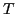, provides strong selection rules for nuclear reactions, decays, and transitions . In particular, the selection rules for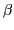-decay Fermi and Gamow-Teller transitions are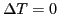and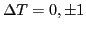, respectively, with the exception of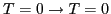transitions that are forbidden [4,5]. The superallowed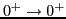Fermi transitions bridge nuclear structure with the electroweak standard model of particle physics, providing the most accurate estimate for the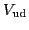matrix element of the CKM matrix [6,7]; hence, testing the CKM unitarity. From a nuclear structure perspective, the unitarity test depends critically on a set of theoretically calculated isospin-breaking corrections whose precise determination poses a challenging problem [8,9,10].

In this work, we calculate the isospin impurities and isospin-breaking corrections to the superallowed Fermi decay by using a newly developed isospin- and angular-momentum-projected DFT approach without pairing [11,12,13]. This technique takes advantage of the ability of the mean field (MF) to properly describe long-range polarization effects. The MF treatment is followed by the isospin projection to remove the unwanted spontaneous isospin mixing within MF [12,14,15,16].

This paper is organized as follows. We begin in Sec. 2 with a short summary of our isospin- and angular-momentum-projected DFT approach. In Sec. 3, we present applications of the isospin-projected DFT variant of the model to the isospin mixing in the ground states (g.s.) of even-even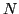=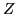nuclei. Section 4 discusses preliminary results for the isospin-breaking corrections to the superallowed beta decays calculated by considering simultaneous isospin and angular-momentum restoration. Finally, the conclusions are contained in Sec. 5.Next: Theory Up: Isospin mixing in nuclei Previous: Isospin mixing in nuclei
Jacek Dobaczewski 2011-02-20